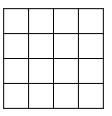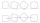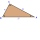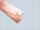# Perimeter from area

What is the perimeter of the square if its content is 64 cm2?

x =  32 cm

### Step-by-step explanation:Did you find an error or inaccuracy? Feel free to write us. Thank you!#### You need to know the following knowledge to solve this word math problem:

We encourage you to watch this tutorial video on this math problem:

## Related math problems and questions:

• WindowsCalculate the masonry area to build a wall with dimensions of 9 m × 4 m with 4 windows of size 64 cm × 64 cm.
• Perimeter to area 2Find the area of a square whose perimeter is 100 cm with solve.
• The playgroundThe playground has the shape of a square with a side of 64 m. It is fenced on three sides. What is the area of the playground and how long is its fence?
• Second sideCalculate the length of the other side of the rectangle if its circumference is 60 cm and one side is 10 cm long.
• Area of squareCalculate the content area of the square whose perimeter is 24 dm.
• Rectangle 45The perimeter of a rectangle is 60cm. If the length of the rectangle is 20cm. a)find the width b)find the area.
• Rectangular trapeziumCalculate the perimeter of a rectangular trapezium when its content area is 576 cm2 and sice a (base) is 30 cm, height 24 cm.
• SquaresCalculate the area of square and result round to square decimeters. a) a = 1,52 dm b) a = 13 268mm c) a = 562 cm d) a = 1,52 m
• HeightThe content of the triangle is 35 cm2. The length of the base is 10 cm. Determine the length of the height on the base.
• CircleHow big is an area of a circle if its circumference is 51.2 cm?
• Do you solve this?Determine area S of rectangle and length of its sides if its perimeter is 102 cm.
• StripFrom 5.9 cm wide strip should be cut rhombus with area 28 cm2. How long will be its side?
• Square s3Calculate the diagonal of the square, where its area is 0.49 cm square. And also calculate its circumference.
• MO-Z5-3-66 tilesThe picture shows a square tiles with side 10 dm which is composed of four identical small rectangles and squares. Circumference of small square is five times smaller than the circumference of the entire tile. Determine the dimensions of the rectangle.
• Isosceles triangle 9Given an isosceles triangle ABC where AB= AC. The perimeter is 64cm, and the altitude is 24cm. Find the area of the isosceles triangle.
• Four circles1) Calculate the circle radius if its area is 400 cm square 2) Calculate the radius of the circle whose circumference is 400 cm. 3) Calculate circle circumference if its area is 400 cm square 4) Calculate the circle's area if perimeter 400 cm.
• Tripled squareIf you tripled the length of the sides of the square ABCD you increases its content by 200 cm2. How long is the side of the square ABCD?